July 17, 2019

# Dynamic Programming

• Similar to divide and conquer
• Solves problems by combining solutions
• Programming refers to solving problems in a tabular way
• Dynamic programming applies when subproblems are not independent
• They share subproblems
• Solves each subproblem only once
• Store the solution to reuse
• Saves time
• Generally used in optimization problems

# Dynamic Programming

• Four steps
1. Characterize the structure of an optimal solution
2. Recursively define the value of an optimal solution
3. Compute the value of an optimal solution in an bottom-up way
4. Construct an optimal solution from the computed information

# The Rod Cutting Problem

• The problem consists in deciding where to cut steel rods
• Serling Enterprises buys long steel rods, cuts them and sells them
• Each cut is free
• What is the best way to cut the rods?
• We know the price $$p_i$$ (in dollars) for rods of $$i$$ inches ($$i$$ is an integer number)

# The Rod Cutting Problem

• Rods prices table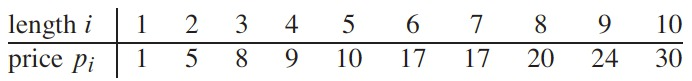# The Rod Cutting Problem

• The rod-cutting problem
• Given a rod of length $$n$$ inches and table of prices $$p_i$$ for $$i = 1, 2, n$$,
• Find the maximum revenue $$r_n$$ obtainable by cutting up the rod and selling the pieces
• A possible solution could be not-cutting the rod at all
• For a rod with $$n = 4$$ and the prices table, the best solution would be cutting the rod into two pices of two inches each and obtaining 10 dollars

# The Rod Cutting Problem

• We can cut a rod of length $$n$$ in $$2^{n-1}$$ different ways
• 8 possible ways to cut a rod of length 4
• i.e a rod cut $$4 = 2 +2$$ shows that a row of length 4 is cut into two pieces of length 2 each
• If an optimal solution cuts the rod into $$k$$ pieces, $$1 \leq k \leq n$$
• $$n = i_1 + i_2 + \dots + i_k$$ provides maximum revenue
• $$r_n = p_{i1} + p_{i2} + \dots + p_{ik}$$.

# The Rod Cutting Problem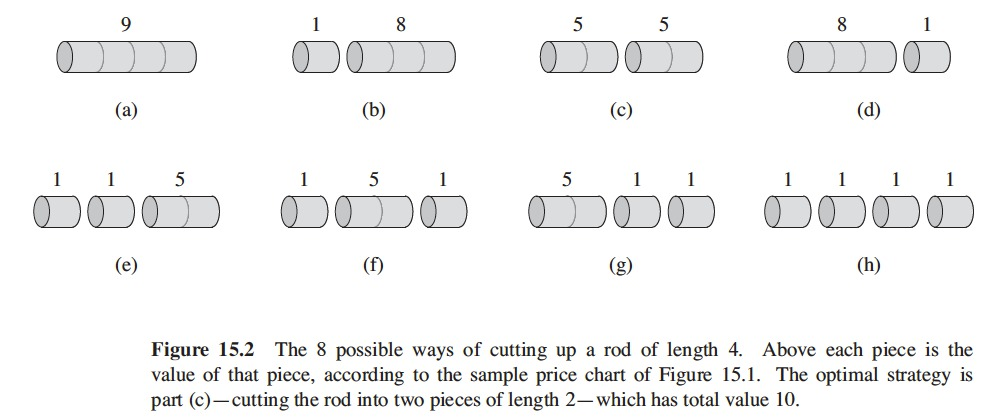# The Rod Cutting Problem

• Optimal revenue figures $$r_i$$ for $$i = 1,2,\dots,10$$ with corresponding optimal decompositions:
• $$r_1 = 1$$ from solution 1 = 1 (no cuts)
• $$r_2 = 5$$ from solution 2 = 2 (no cuts)
• $$r_3 = 8$$ from solution 3 = 3 (no cuts)
• $$r_4 = 10$$ from solution 4 = 2 + 2
• $$r_5 = 13$$ from solution 5 = 2 + 3
• $$r_6 = 17$$ from solution 6 = 6 (no cuts)
• $$r_7 = 18$$ from solution 7 = 1 + 6 or 7 = 2 + 2 + 3
• $$r_8 = 22$$ from solution 8 = 2 + 6
• $$r_9 = 25$$ from solution 9 = 3 + 6
• $$r_{10} = 30$$ from solution 10 = 10 (no cuts)

# The Rod Cutting Problem

• More generally
• $$r_n = \max(p_n, r_1 + r_{n-1}, r_2+r_{n-2}, \dots, r_{n-1} + r_1)$$
• $$p_n$$, making no cuts at all and selling the rod uncut
• $$r_i + r_{n-i}$$, initial cut of size $$i$$ and other of size $$n-i$$, for each $$i = 1, 2, \dots, n-1$$

# The Rod Cutting Problem

• To solve the problem of original size $$n$$
• We solve smaller problems of same type but smaller sizes
• Rod-cutting problem exhibits optimal substructure
• Optimal solutions to a problem incorporate optimal solutions to related subproblems, which we may solve independently

# The Rod Cutting Problem

• Another way to view the decomposition,
• First piece of length $$i$$ cut off, left-hand end
• Right-hand remainder of length $$n-i$$
• The remainder may be further divided
• A first piece followed by some decomposition of the remainder
• $$r_n = \max_{1 \leq i \leq n} (p_i + r_{n-i})$$
• When no-cuts at all: first piece has size $$i = n$$ and revenue $$p_n$$ and remainder has size 0 with revenue $$r_0=0$$
• This formulation embodies the solution to only one related subproblem
• The remainder, instead of two

# The Rod Cutting Problem

• Recursive top-down implementation
• Input: array $$p[1 .. n]$$ of prices and integer $$n$$, the rod length
• Output: maximum revenue possible for a rod of length $$n$$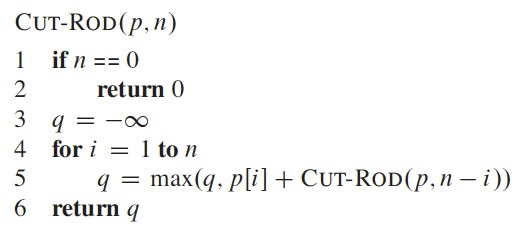# The Rod Cutting Problem

• The recursive implementation takes a long time to run for moderate size inputs (i.e. $$n = 40$$, several minutes to more than an hour)
• It calls itself with the same parameters many times
• Solves the same problem repeatedly

# The Rod Cutting Problem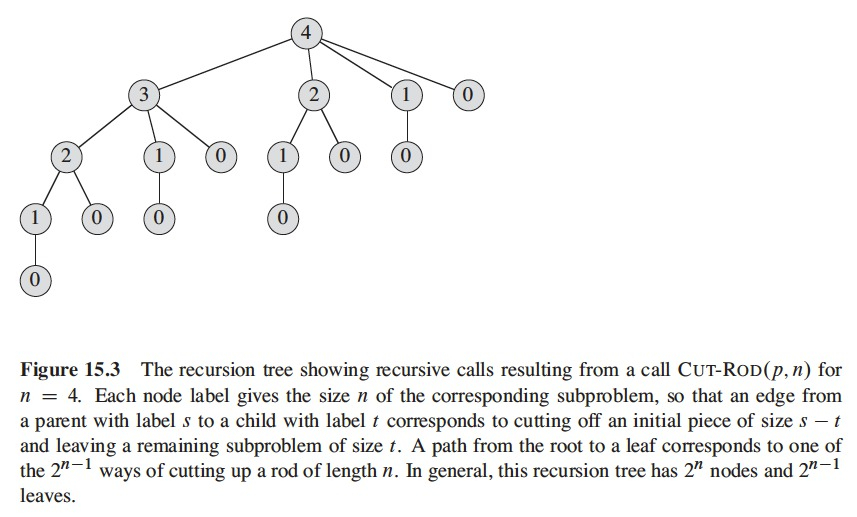# The Rod Cutting Problem

• Running time of CUT-ROD
• $$T(n)$$ denotes number of calls made to CUT-ROD when called with second parameter equal to $$n$$
• This is the number of nodes in a subtree with root at $$n$$ in the recursion tree
• Includes initial call at its root so $$T(0) = 1$$
• $$T(n) = 1 + \sum_{j=0}^{n-1} T(j)$$.
• 1 for call at root node
• $$T(j)$$ counts number of calls (including recursive) due to CUT-ROD($$p, n-i$$), where $$j=n-i$$.
• Solving the recurrence we find that $$T(n) = 2^n$$, exponential in $$n$$

# The Rod Cutting Problem

• ROD-CUT explores the $$2^{n-1}$$ possible ways of cutting up a rod of length $$n$$
• The recursion tree has $$2^{n-1}$$ leaves

# The Rod Cutting Problem

• Using Dynamic Programming for Optimal Cutting
• Naive recursive solution is inefficient, we want CUT-ROD to be more efficient
• We want each subproblem to be solved only once
• We save the solution of those subproblems
• We just look for those solutions instead of recomputing them
• Dynamic programming uses additional memory
• Savings may be really good
• i.e. exponential $$\rightarrow$$ polynomial
• Number of distinct subproblems is polynomial in the input size
• Those problems can be solved in polynomial time

# The Rod Cutting Problem

• Two ways to implement dynamic programming
• Top-down with memoization
• Recursive procedure
• Modified to save results to each subproblem (in array or hash table)
• Procedure checks if subproblem has been solved previously
• If previously solved, it gets the value
• If it hasn’t been previously solved, y computes its value
• The recursive procedure has been memoized, it remembers previous previous results

# The Rod Cutting Problem

• Two ways to implement dynamic programming
• Bottom-up method
• Depends on natural notion of size of a subproblem
• Solving a subproblem depends only on solving smaller subproblems
• Sort problems by size and solve them in size order, smaller first
• When we solve a subproblem, all smaller subproblems used to compute its solution have been solved
• Solutions are stored and computed only once

# The Rod Cutting Problem

• These approaches yield algorithms with the same asymptotic running time
• Except when top-down approach doesn’t actually recurse to examine all possible subproblems
• Bottom-up approach has better constant factors
• Less overhead for procedure calls

# The Rod Cutting Problem

• Memoized Cut Rod Version
• $$p$$ holds the list of prices
• $$n$$ is the size of the rod
• $$r$$ is an array to store the solutions to subproblems
• $$- \infty$$ means that subproblem hasn’t been solved yet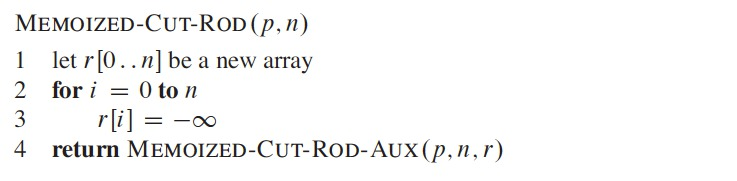# The Rod Cutting Problem

• Lines 1-2 checks if desired value has already been computed and returns it
• Lines 3-7 compute the new value (previously unknown)
• Line 8 saves the computed value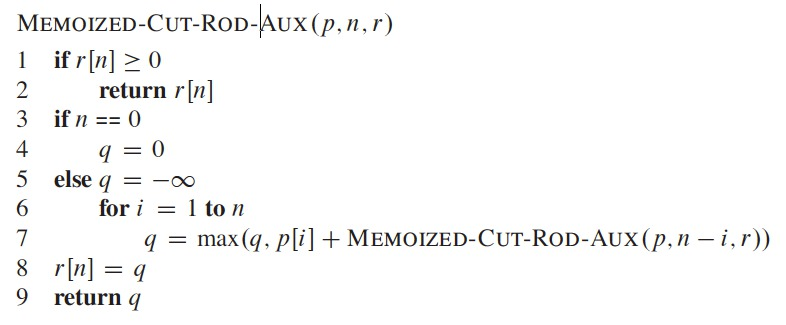# The Rod Cutting Problem

• Bottom-up Cut Rod Version
• Stores subproblem solutions in $$r$$
• Solves subproblems of size $$j$$, in order of increasing size: $$j=1,2, \dots, n$$
• In line 6 the algorithm directly references array entry $$r[j-i]$$
• Instead of making a recursive call to solve subproblem of size $$j-i$$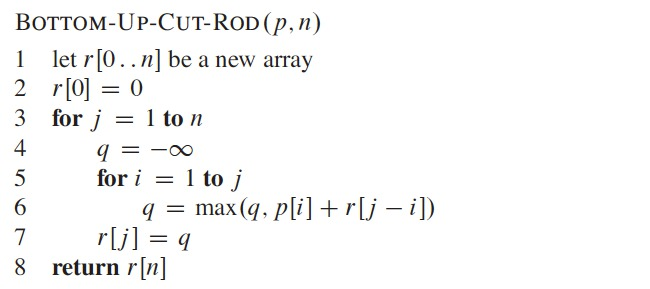# The Rod Cutting Problem

• Running time of the memoized and bottom-up of the CUT-ROD algorithm
• $$\Theta(n^2)$$ for both of them
• BOTTOM-UP-CUT-ROD
• Doubly-nested loop structure
• Iterations of inner for loop (lines 5-6) forms an arithmetic series
• Then, it runs in $$\Theta(n^2)$$
• MEMOIZED-CUT-ROD
• Recursive call to solve previously solved subproblem returns immediately
• It solves a subproblem only once
• Total number of iterations for loop of lines 6-7, is $$n$$
• Forms an arithmetic series for a total of $$\Theta(n^2)$$

# The Rod Cutting Problem

• The arithmetic series (from the textbook)
• The summation $$\sum\limits_{k=1}^{n} k = 1 + 2 + \dots + n$$
• Is an arithmetic series and has the value
• $$\sum\limits_{k=1}^{n} k = \frac{1}{2} n(n+1) = \Theta(n^2)$$.

# The Rod Cutting Problem

• Subproblem graphs
• Subproblems involved and how subproblems depend on one another
• Directed graph, one vertex for each distinct subproblem
• Like a collapsed version of the recursion tree for the top-down recursive method
• Bottom-up dynamic programming algorithm
• Consider vertices in an order: reverse topological sort of the subproblem graph
• No subproblem is considered until all subproblems that it depends upon have been solved
• Size of the subproblem graph $$G=(V,E)$$ help us determine running time of the dynamic programming algorithm
• Sum running times of each different subproblem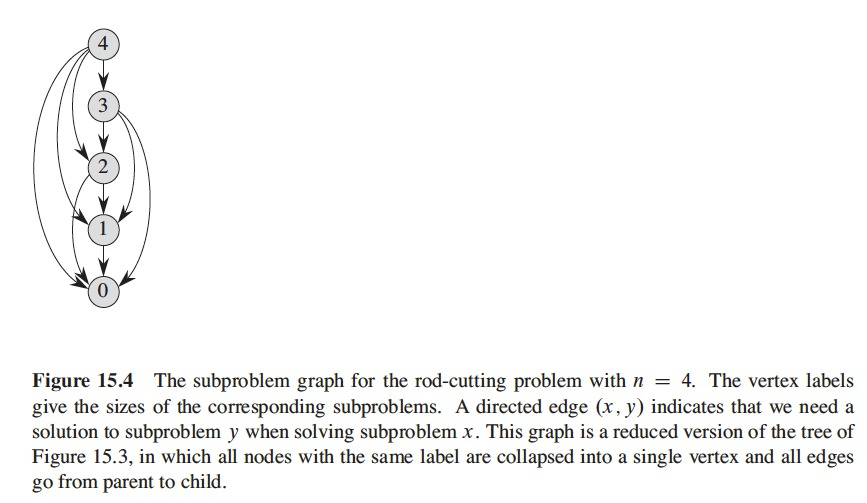# The Rod Cutting Problem# The Rod Cutting Problem

• Reconstructing a solution
• Our Dynamic Programming (DP) algorithm
• Returns value of an optimal solution
• Doesn’t return an actual solution: a list of piece sizes
• Extend the DP approach to also record a choice that led to the optimal value
• With this information, we can print an optimal solution

# The Rod Cutting Problem

• BOTTOM-UP-CUT-ROD, extended
• Computes for each rod size $$j$$: maximum revenue $$r_j$$ and optimal size of first piece to cut off $$s_j$$
• In line 8,
• We store in $$s[j]$$ the optimal size $$i$$ of the first piece to cut off when solving problem of size $$j$$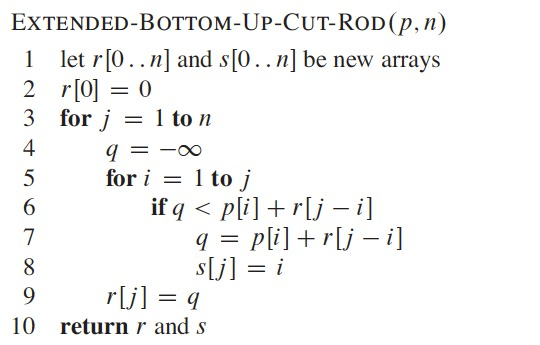# The Rod Cutting Problem

• Printing the solution
• Need price table $$p$$ and a rod size $$n$$
• Calls EXTENDED-BOTTOM-UP-CUT-ROD to compute $$s[1 .. n]$$
• Prints out the list of pieces sizes for an optimal decomposition of a rod of length $$n$$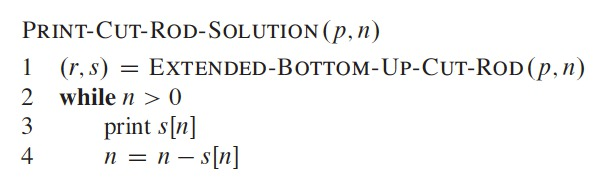# The Rod Cutting Problem

• Call to EXTENDED-BOTTOM-UP-CUT-ROD($$p$$,10)
• Returns the arrays
• Call to PRINT-CUT-ROD-SOLUTION($$p$$,10) returns just 10
• Call to PRINT-CUT-ROD-SOLUTION($$p$$,7) returns 1 and 6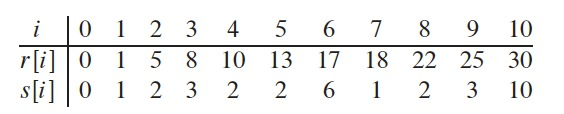# Matrix-chain Multiplication

• Given a sequence (chain) $$\langle A_1, A_2, \dots, A_n \rangle$$ of $$n$$ matrices
• Compute the product $$A_1 A_2 \dots A_n$$
• Matrix multiplication is associative
• All parenthesizations yield the same product
• Fully parenthesized product
• Either a single matrix
• Product of two fully parenthesized matrix products, surrounded by parentheses

# Matrix-chain Multiplication

• Fully parenthesized product of $$A_1 A_2 A_3 A_4$$:
• $$(A_1(A_2(A_3 A_4)))$$,
• $$(A_1((A_2 A_3)A_4))$$,
• $$((A_1 A_2)(A_3 A_4))$$,
• $$((A_1(A_2 A_3))A_4)$$,
• $$(((A_1 A_2)A_3)A_4)$$.
• Way of parenthesization has dramatic impact on cost of evaluating the product

# Matrix-chain Multiplication

• Standard pseudocode (from SQUARE-MATRIX-MULTIPLY procedure, section 4.2)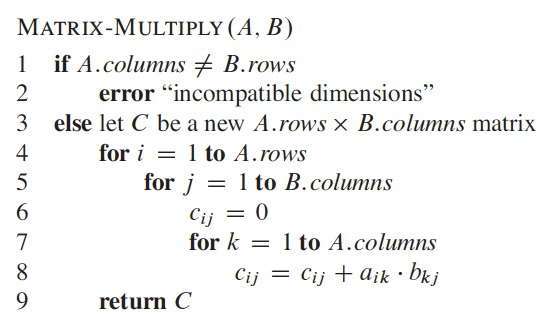# Matrix-chain Multiplication

• We require $$A$$ and $$B$$ to be compatible
• $$A$$ is a $$p \times q$$ matrix, $$B$$ then is a $$q \times r$$ matrix
• The result $$C$$ is an $$p \times r$$ matrix
• Time to compute $$C$$ dominated by scalar multiplications (line 8) as $$pqr$$
• We will express costs as number of scalar multiplications

# Matrix-chain Multiplication

• Different costs by different parenthesizations of a matrix product
• Chain of 3 matrices $$\langle A_1, A_2, A_3 \rangle$$
• Dimensions: $$10 \times 100$$, $$100 \times 5$$, and $$5 \times 50$$
• $$((A_1 A_2)A_3)$$
• We perform $$10 \cdot 100 \cdot 5 = 5000$$ scalar multiplications to get $$A_1 A_2$$
• We perform $$10 \cdot 5 \cdot 50 = 2500$$ scalar multiplications to get the final result
• Total of 7,500 scalar multiplications
• $$(A_1(A_2 A_3))$$
• We perform $$100 \cdot 5 \cdot 50 = 25,000$$ scalar multiplications to get $$A_2 A_3$$
• We perform $$10 \cdot 100 \cdot 50 = 50,000$$ scalar multiplications to get the final result
• Total of 75,000 scalar multiplications

# Matrix-chain Multiplication

• Matrix-chain multiplication problem
• “Given a chain $$\langle A_1, A_2, \dots, A_n \rangle$$ of $$n$$ matrices, for $$i = 1, 2, \dots, n$$, matrix $$A_i$$ has dimension $$p_{i-1} \times p_i$$, fully parenthesize the product $$A_1 A_2 \dots A_n$$ in a way that minimizes the number of scalar multiplications.”
• Goal: find the order of multiplying matrices with the lowest cost

# Matrix-chain Multiplication

• Counting the number of parenthesizations
• $$P(n)$$ is the number of alternative parenthesizations of sequence of $$n$$ matrices
• $$n=1$$,we have 1 matrix and only 1 way to fully parenthesize
• $$n \geq 2$$, a product is the product of 2 fully parenthesized matrix subproducts
• Split may occur between the $$k^{th}$$ and $$(k+1)$$ matrices for $$k=1,2, \dots, n-1$$
• We obtain recurrence 15.6, with solution $$\Omega(2^n)$$
• There is an exponential number of parenthesizations $$\Omega(2^n)$$, exponential in $$n$$
• Brute force algorithm performs poorly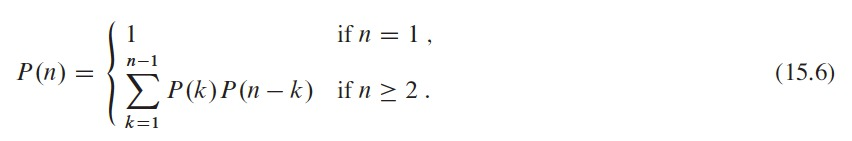# Matrix-chain Multiplication

• Applying dynamic programming
• Optimally parenthesize a matrix chain
• Four steps
1. Characterize the structure of an optimal solution
2. Recursively define the value of an optimal solution
3. Compute the value of an optimal solution
4. Construct an optimal solution from computed information

# Matrix-chain Multiplication

• Step 1: The structure of an optimal parenthesization
• Let $$A_{i,j}$$ be the matrix resulting from product $$A_i A_{i+1} \dots A_j$$ with $$i < j$$
• If we divide the product between $$A_k$$ and $$A_{k+1}$$ for $$i \leq k < j$$
• We then compute $$A_{i..k}$$ and $$A_{k+1..j}$$ separately
• Solution for each subproblem must be optimal in order to make solution of $$A_1 .. A_n$$ optimal
• Cost = Cost($$A_{i..k}$$) + Cost($$A_{k+1..j}$$) + Cost for multiplying both matrices
• If there were another way to group the matrices with better cost, then this wouldn’t be optimal

# Matrix-chain Multiplication

• Step 1: The structure of an optimal parenthesization
• We need to find the best way to split the product, $$k$$
• We use this optimal substructure to construct an optimal solution from optimal solution to subproblems
• We split the product into two subproblems, $$A_i A_{i+1} \dots A_k$$ and $$A_{k+1} A_{k+2} \dots A_j$$
• When we search for correct place to split the product, we consier all possible places, to make sure we find the optimal one

# Matrix-chain Multiplication

• Step 2: A recursive solution
• We define the cost of an optimal solution
• Recursively in terms of the optimal solution to subproblems
• Subproblems
• Problem of determining the minimum cost of grouping the matrices with parenthesis for $$A_i A_{i+1} \dots A_j$$ for $$1 \leq i \leq j \leq n$$
• Let $$m[i,j]$$ be the minimum number of scalar multiplications to compute $$A_{i,j}$$
• The total cost to get $$A_{1..n}$$ would be $$m[1,n]$$

# Matrix-chain Multiplication

• Step 2: A recursive solution
• We define $$m[i,j]$$
• If $$i = j, m[i,j] = 0$$, trivial problem, only one matrix, no need for scalar multiplications
• If $$i < j$$, we assume optimal division between $$A_k$$ and $$A_{k+1}$$, ($$i \leq k < j$$)
• $$m[i,j]$$ = cost to compute $$A_{i,k}$$ + cost to compute $$A_{k+1,j}$$ + cost to compute $$A_{i..k}A_{k+1..j}=m[i,k] + m[k+1,j] + p_{i-1}p_kp_j$$
• But we don’t know the value of $$k$$ and we will have to try all $$j-i$$ possibilities

# Matrix-chain Multiplication

• Step 2: A recursive solution
• The recursive definition for the minimum cost to group the parenthesis of the product $$A_iA_{i+1} \dots A_j$$ is shown in equation 15.7
• We still need to define $$s[i,j]$$ as the value of $$k$$ in which we split the product $$A_iA_{i+1} \dots A_j$$ in an optimal parenthesization
• $$s[i,j]$$ is the value of $$k$$ such that $$m[i,j] = m[i,k] + m[k+1,j] + p_{i-1}p_kp_j$$.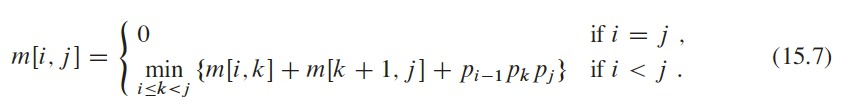# Matrix-chain Multiplication

• Step 3: Computing the optimal cost
• We can use the recurrence to compute the minimum cost of $$m[1,n]$$ to multiply $$A_1A_2 \dots A_n$$
• But the algorithm is still exponential (no better than brute force)
• But we can take advantage that we have relatively few subproblems
• One for each selection of $$i$$ and $$j$$ where $$1 \leq i \leq j \leq n$$, or
• $${n \choose 2} + n = \Theta(n^2)$$
• The algorithm can find repeated subproblems
• Overlapping
• We use a bottom-up tabular method

# Matrix-chain Multiplication

• Step 3: Computing the optimal cost
• Bottom-up method
• We solve smaller subproblems first and the largest ones will be easier to solve
• We define 2 arrays
• $$m[1..n, 1..n]$$, to store the minimum costs of multiplying $$A_i \dots A_j$$
• $$m[1,n]$$ stores the cost of multiplying $$A_1 A_2 \dots A_n$$
• $$s[1..n, 1..n]$$, to store the optimal divisions, the index of $$k$$
• Used to construct the optimal solution

# Matrix-chain Multiplication

• Step 3: Computing the optimal cost
• Execution time of $$O(n^3)$$ and requires $$\Theta(n^2)$$ memory to store $$m$$ and $$s$$
• Optimal substructure + Overlapping subproblems $$\rightarrow$$ dynamic programming applies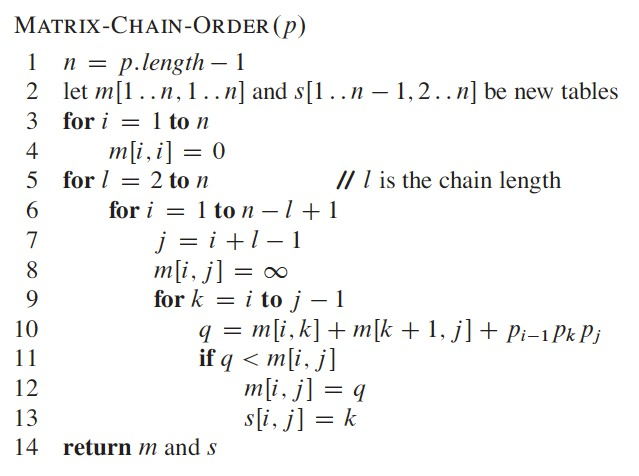# Matrix-chain Multiplication

• Step 3: Computing the optimal cost
• First computes $$m[i,i] = 0$$ for $$i = 1,2, \dots n$$
• First execution of for loop in lines 5-13
• Computes $$m[i,i+1]$$ for $$i = 1,2, \dots, n-1$$ (minimum costs for chains of length $$l=2$$)
• Second execution of for loop in lines 5-13
• Computes $$m[i,i+2]$$ for $$i = 1,2, \dots, n-2$$ (minimum costs for chains of length $$l=3$$)
• At all times the $$m[i,j]$$ cost computed in lines 10-13 depends only on table entries $$m[i,k]$$ and $$m[k+1,j]$$ already computed

# Matrix-chain Multiplication

• Step 3: Computing the optimal cost
• Procedure on a chain of $$n = 6$$ matrices
• $$m[i,j]$$ defined for $$i \leq j$$, only use portion of $$m$$ above the diagonal
• Matrix chain listed at the bottom

# Matrix-chain Multiplication

• Step 3: Computing the optimal cost